GATE  >  Test: Surveying- 1

# Test: Surveying- 1

Test Description

## 10 Questions MCQ Test GATE Civil Engineering (CE) 2023 Mock Test Series | Test: Surveying- 1

Test: Surveying- 1 for GATE 2023 is part of GATE Civil Engineering (CE) 2023 Mock Test Series preparation. The Test: Surveying- 1 questions and answers have been prepared according to the GATE exam syllabus.The Test: Surveying- 1 MCQs are made for GATE 2023 Exam. Find important definitions, questions, notes, meanings, examples, exercises, MCQs and online tests for Test: Surveying- 1 below.
Solutions of Test: Surveying- 1 questions in English are available as part of our GATE Civil Engineering (CE) 2023 Mock Test Series for GATE & Test: Surveying- 1 solutions in Hindi for GATE Civil Engineering (CE) 2023 Mock Test Series course. Download more important topics, notes, lectures and mock test series for GATE Exam by signing up for free. Attempt Test: Surveying- 1 | 10 questions in 30 minutes | Mock test for GATE preparation | Free important questions MCQ to study GATE Civil Engineering (CE) 2023 Mock Test Series for GATE Exam | Download free PDF with solutions
 1 Crore+ students have signed up on EduRev. Have you?
Test: Surveying- 1 - Question 1

### The sensitiveness of a bubble tube in a level would decrease if

Detailed Solution for Test: Surveying- 1 - Question 1

Sensitivity of a bubble tube/ level tubs is its capability of exhibiting small deviation of the tube horizontally.

Sensitivity increases when:
Temperature Decreases
Larger diameter
Larger Length
Higher length of one division
Higher smoothness of finish of internal surface of liquid
Low surface tension and viscosity

Test: Surveying- 1 - Question 2

### Hydrographic surveys deal with the mapping of

Detailed Solution for Test: Surveying- 1 - Question 2

Types of survey on the basis of place
Land Survey: Done on Land
Hydrographic Survey: Survey of water bodies like streams, sea, ponds etc.
Underground Survey: required for construction of tunnels.
Aerial Survey: Aerial photographs via aeroplanes, drones etc. Preparation of large scale maps of an area.

Test: Surveying- 1 - Question 3

### If "L" is the length of the chain , "W" is the weight of the chain and 'T' is the tension , the Sag Correction for the chain line is

Detailed Solution for Test: Surveying- 1 - Question 3

When tape is supported at two ends then it will always sag downwards due to its own weight, thus, apparent length larger than the given length. The correction is always negative and is given by-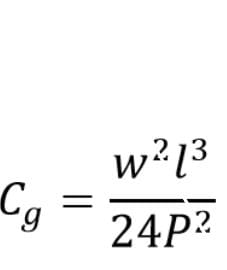C_g=(w2 l3)/(24P2 )
w weight per unit length
P applied pull
l  length of tape suspended between the supports.

Test: Surveying- 1 - Question 4

If the whole circle bearing is 315°20′, its quadrantal bearing would be

Detailed Solution for Test: Surveying- 1 - Question 4

As it can be seen from the figure the quadrantal bearing is N44°40′W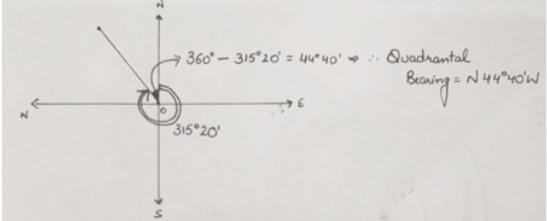*Answer can only contain numeric values
Test: Surveying- 1 - Question 5

The radius of a 1 degree curve is __________ m

Detailed Solution for Test: Surveying- 1 - Question 5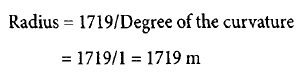Test: Surveying- 1 - Question 6

A level when set up 25 m from peg A and 50 m from peg B reads 2.847 on a staff held on A and 3.462 on a staff held on B, keeping bubble at its centre while reading. If the reduced levels of A and B are 283.665 m and 284.295 m respectively, the collimation error per 100 m is

Detailed Solution for Test: Surveying- 1 - Question 6

Let the collimation error per 100m is e
Correction for staff reading at A = (25/100)e= 0.25e
Correction for staff reading at B = (50/100)e =0.5e
True staff reading at A = Observed Value + Correction = 2.847+0.25e
True staff reading at B = 3.462+0.5e
Difference in elevation = RLa – RLb =283.665-284.295= -0.63m
=2.847+0.25e-(3.462+0.5e) = -0.615-0.25e =-0.63

e = 0.06m

Test: Surveying- 1 - Question 7

An invar tape, 50 m in length, standardized at 20oC temperature and 10kg pull, is used to measure a baseline. The Correction per tape length, if at the time of measurement the temperature was 30o and the coefficient of linear expansion of the tape was 1x10-6 per oC , will be

Detailed Solution for Test: Surveying- 1 - Question 7

Correction per tape length is given by: (Coefficient of Linear Expansion) x (Change in Temperature) x (Tape Length)
= 10-6 x (30-20) x 50 = 0.0005 m

Test: Surveying- 1 - Question 8

A Rectangular plot of 16km2  in area is shown on a map by a similar rectangular area of 1 cm2 , R.F of the scale to measure a distance of 40km will be

Detailed Solution for Test: Surveying- 1 - Question 8

Representative fraction of the map (in terms of length) =√((Area on Map)/(Actual Area of plot))
Area on Map = 1 cm2 = 1 x 10-6 m2
Actual Area of plot = 16 km2 = 16 x 106 m2
Representative fraction of the map (in terms of length) = √(〖10〗(-6)/(16×〖10〗6 )) =1/4000000

Test: Surveying- 1 - Question 9

Following observations were taken with a transit fitted with stadia wires.
The line of sight was horizontal and the staff was held vertical.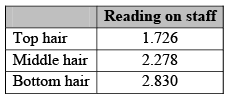The tacheometric constants k and C are 100 and 0.4 m, respectively. The horizontal distance between staff and instrument is

Detailed Solution for Test: Surveying- 1 - Question 9

D = KS + C

S  = 2.830 - 1.726 = 1.104

D = 100 x 1.104 + 0.4 = 110.8m

Test: Surveying- 1 - Question 10

Following observations were taken during a reciprocal leveling: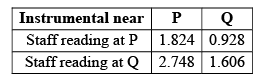If reduced level of P is 140.815 m, the reduced level of Q is

Detailed Solution for Test: Surveying- 1 - Question 10

Explanation : True difference = 1.824-0.928/2.748-1.606 = 0.7845

Reduced level of Q is = 140.815-0.7845

= 140.030m

## GATE Civil Engineering (CE) 2023 Mock Test Series

26 docs|292 tests
 Use Code STAYHOME200 and get INR 200 additional OFF Use Coupon Code
Information about Test: Surveying- 1 Page
In this test you can find the Exam questions for Test: Surveying- 1 solved & explained in the simplest way possible. Besides giving Questions and answers for Test: Surveying- 1, EduRev gives you an ample number of Online tests for practice

## GATE Civil Engineering (CE) 2023 Mock Test Series

26 docs|292 tests